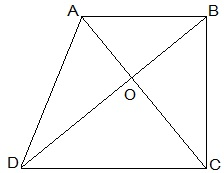# Diagonals AC and BD of a quadrilateral ABCD intersect at O in such a way that ar(△AOD) = ar(△BOC) . Prove that ABCD is a trapezium.Solution

Given:

ar(ΔAOD) = ar(ΔBOC)

Prove:

ABCD is a trapezium.

Proof:

ar(ΔAOD) = ar(ΔBOC)

=> ar(ΔAOD) + ar(ΔAOB) = ar(ΔBOC)+ar(ΔAOB)

Areas of ΔADB and ΔACB are equal.

Thus

They should be lying between the same parallel lines.

Hence AB ∥ CD

Therefore, ABCD is a trapezium.

Hence Proved